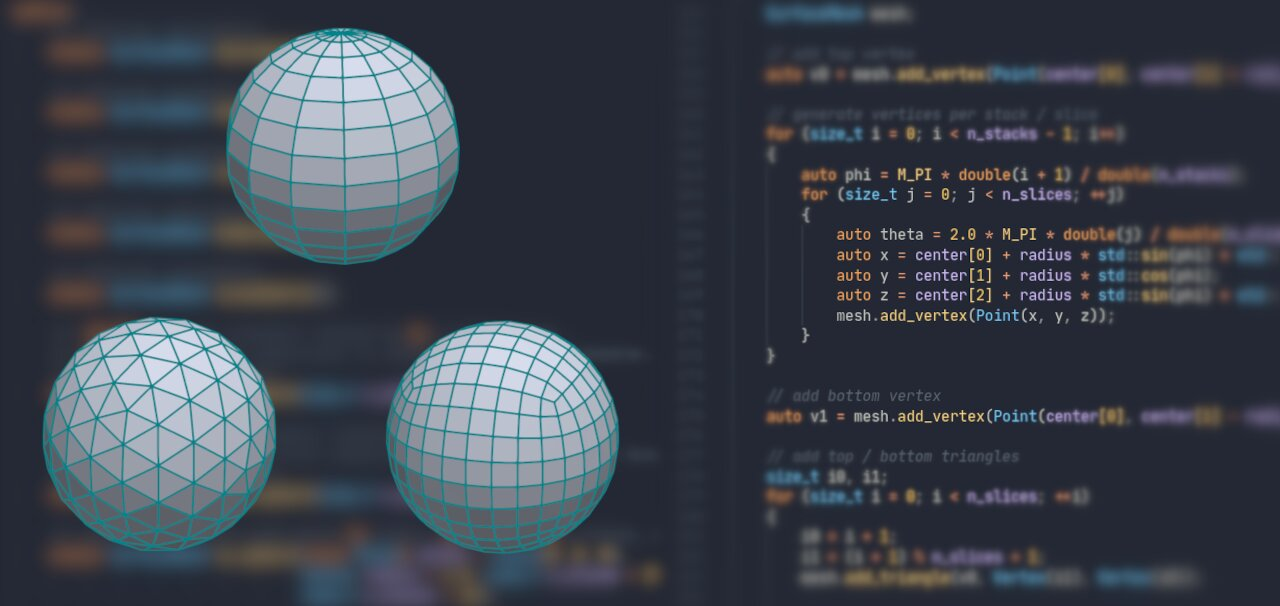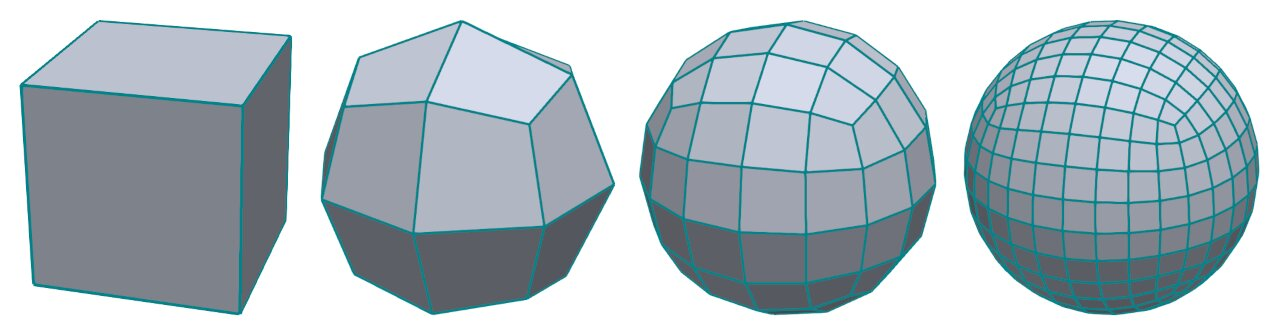# Generating Meshes of a SphereSpheres are one of the most basic geometric shapes we can think of. However, there are a variety of methods for generating surface meshes of a sphere. In this brief tutorial, I will describe four of them:

1. UV sphere
2. Icosphere
4. Goldberg polyhedra

This article is a follow-up on my previous tutorial on generating Platonic solids. I recommend reading it first since I will re-use parts of the code from that article. The code is in C++ and using the PMP library. Use the PMP project template for ease of implementation and visualization.

## The UV SphereThe UV sphere is a standard shape available in many 3D modeling tools such as Blender. The basic approach is to tessellate the sphere along meridians and parallels, i.e., lines from one pole to another and lines parallel to the equator. The resulting mesh contains a fan of triangles around the poles and quadrilateral elements everywhere else.

The code below is rather straightforward. I first add all vertices and then add the corresponding triangular and quadrilateral faces. Note that I intentionally did not optimize the code in any way for the purpose of this article.

``````SurfaceMesh uv_sphere(int n_slices, int n_stacks)
{
SurfaceMesh mesh;

auto v0 = mesh.add_vertex(Point(0, 1, 0));

// generate vertices per stack / slice
for (int i = 0; i < n_stacks - 1; i++)
{
auto phi = M_PI * double(i + 1) / double(n_stacks);
for (int j = 0; j < n_slices; j++)
{
auto theta = 2.0 * M_PI * double(j) / double(n_slices);
auto x = std::sin(phi) * std::cos(theta);
auto y = std::cos(phi);
auto z = std::sin(phi) * std::sin(theta);
}
}

auto v1 = mesh.add_vertex(Point(0, -1, 0));

// add top / bottom triangles
for (int i = 0; i < n_slices; ++i)
{
auto i0 = i + 1;
auto i1 = (i + 1) % n_slices + 1;
i0 = i + n_slices * (n_stacks - 2) + 1;
i1 = (i + 1) % n_slices + n_slices * (n_stacks - 2) + 1;
}

for (int j = 0; j < n_stacks - 2; j++)
{
auto j0 = j * n_slices + 1;
auto j1 = (j + 1) * n_slices + 1;
for (int i = 0; i < n_slices; i++)
{
auto i0 = j0 + i;
auto i1 = j0 + (i + 1) % n_slices;
auto i2 = j1 + (i + 1) % n_slices;
auto i3 = j1 + i;
Vertex(i2), Vertex(i3));
}
}
return mesh;
}
``````

## The IcosphereAn alternative to the classic UV sphere is the icosphere. The core idea here is to iteratively subdivide an icosahedron. In contrast to the UV sphere, the resulting mesh is a pure triangle mesh and has a much more regular vertex distribution and element size.

Generating an icosphere basically requires three steps:

1. Generate an initial icosahedron
2. Subdivide the triangles
3. Project the vertices to the sphere

I already covered steps #1 and #3 in my previous article. We can directly re-use the `icosahedron()` and `project_to_unit_sphere()` functions.

The only missing piece is a way to subdivide the triangles of the initial icosahedron. In this implementation, I’m using the Loop subdivision function available in PMP. See the reference docs and the Wikipedia article for details.

The resulting code is straightforward:

``````SurfaceMesh icosphere(size_t n_subdivisions)
{
auto mesh = icosahedron();
SurfaceSubdivision subdivision(mesh);
for (size_t i = 0; i < n_subdivisions; i++)
{
subdivision.loop();
project_to_unit_sphere(mesh);
}
return mesh;
}
``````

Bonus task: Try implementing the Loop subdivision step yourself! The algorithm is rather straightforward and provides a excellent opportunity to exercise your skills to work with meshes. If you get stuck, you can always check the implementation provided in PMP.The quad sphere is another standard shape available in many 3D modeling tools. The algorithm to generate a quad sphere iteratively subdivides an initial hexahedron. As the name implies, the resulting mesh is a pure quad mesh.

The code is quite similar to the `icosphere()` function above: I start by generating a hexahedron and then use a subdivision function to refine the mesh. As before, I project the points back to the unit sphere during each iteration to get a nicely regularized shape.

``````SurfaceMesh quad_sphere(size_t n_subdivisions)
{
auto mesh = hexahedron();
SurfaceSubdivision subdivision(mesh);
for (size_t i = 0; i < n_subdivisions; i++)
{
subdivision.catmull_clark();
project_to_unit_sphere(mesh);
}
return mesh;
}
``````

I already introduced the `hexahedron()` and `project_to_unit_sphere()` functions before. However, since I’m dealing with a quad mesh here, I need something different for the subdivision step. The algorithm of choice is Catmull-Clark subdivision, a very well known technique in computer graphics. See the PMP reference docs and the Wikipedia article for more information.

## Goldberg PolyhedraYou can generate yet another type of sphere mesh simply by re-using another function already introduced in the previous article. The `dual()` function computes the dual polyhedron of a mesh. Applying this function to the icosphere meshes yields polygonal meshes as shown above, also known as Goldberg polyhedra. The resulting meshes contain only hexagonal and pentagonal faces.

## Wrapping Up

That’s all for today. I said it would be a brief article, and so it is. Hope this was useful anyway. The full code is available to experiment with as well.